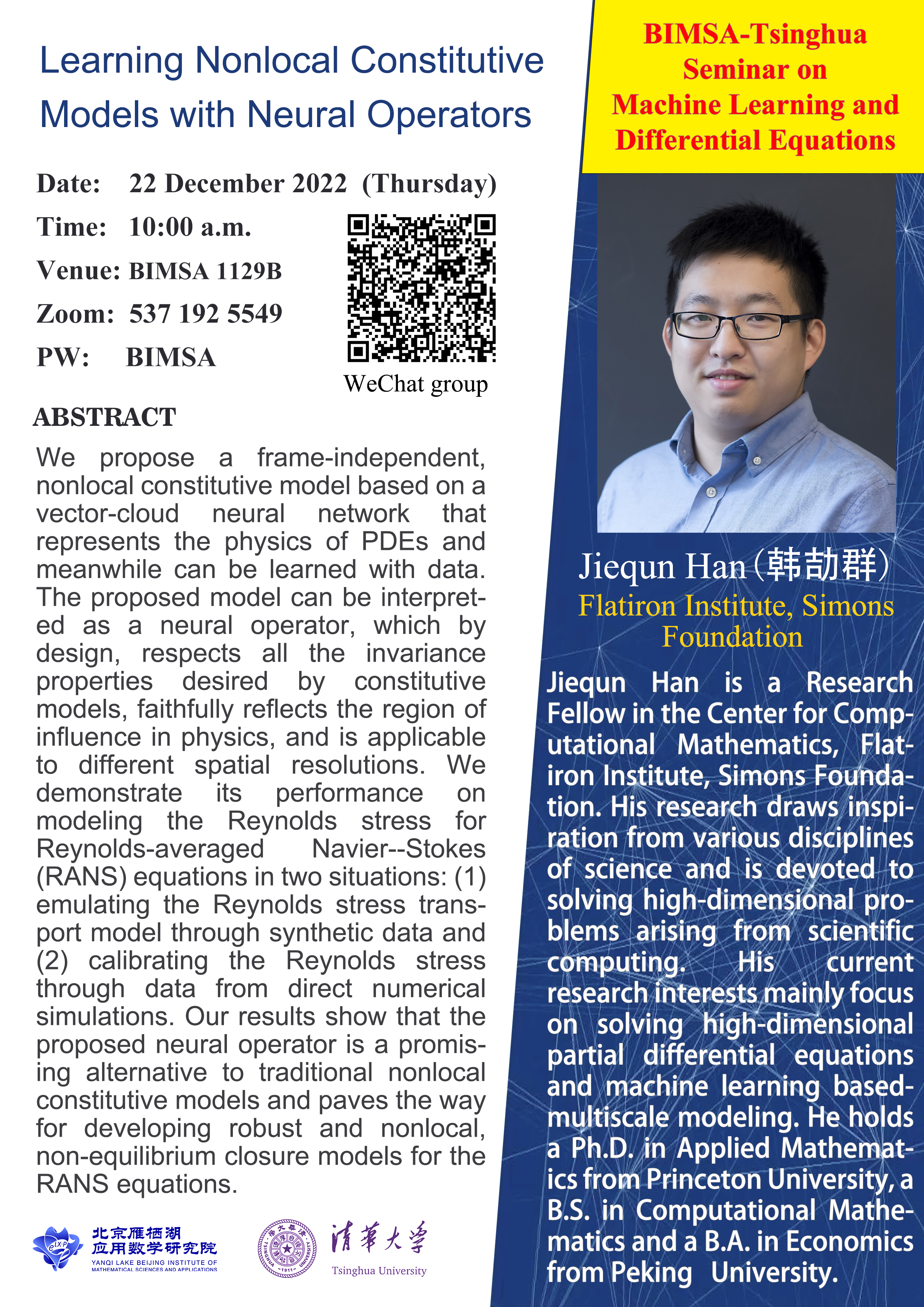# Learning Nonlocal Constitutive Models with Neural Operators

Speaker:     Jiequn Han (Flatiron Institute, Simons Foundation)

Date:    2022.12.12

Time:   10:00 am

Venue: BIMSA 1129B

Zoom:  537 192 5549     Passcode: BIMSA

Video

Abtract：

Constitutive models are widely used for modeling complex systems in science and engineering, when first-principle-based, well-resolved simulations are prohibitively expensive. For example, in fluid dynamics, constitutive models are required to describe nonlocal, unresolved physics such as turbulence and laminar-turbulent transition. However, traditional constitutive models based on PDEs often lack robustness and are too rigid to accommodate diverse calibration datasets. We propose a frame-independent, nonlocal constitutive model based on a vector-cloud neural network that represents the physics of PDEs and meanwhile can be learned with data. The proposed model can be interpreted as a neural operator, which by design, respects all the invariance properties desired by constitutive models, faithfully reflects the region of influence in physics, and is applicable to different spatial resolutions. We demonstrate its performance on modeling the Reynolds stress for Reynolds-averaged Navier--Stokes (RANS) equations in two situations: (1) emulating the Reynolds stress transport model through synthetic data and (2) calibrating the Reynolds stress through data from direct numerical simulations. Our results show that the proposed neural operator is a promising alternative to traditional nonlocal constitutive models and paves the way for developing robust and nonlocal, non-equilibrium closure models for the RANS equations.

Speaker Intro.

Jiequn Han is a Research Fellow in the Center for Computational Mathematics, Flatiron Institute, Simons Foundation. His research draws inspiration from various disciplines of science and is devoted to solving high-dimensional problems arising from scientific computing. His current research interests mainly focus on solving high-dimensional partial differential equations and machine learning based-multiscale modeling. He holds a Ph.D. in Applied Mathematics from Princeton University, a B.S. in Computational Mathematics and a B.A. in Economics from Peking University.[BIMSA-Tsinghua Seminar on Machine Learning and Differential Equations]